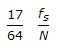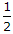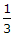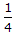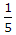# Civil Engineering - Theory of Structures

Exercise : Theory of Structures - Section 4
26.
A close coil helical spring of mean diameter D consists of n coils of diameter d. If it carries an axial load W, the energy stored in the spring, is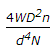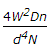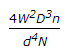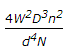Explanation:
No answer description is available. Let's discuss.

27.
A simply supported beam A carries a point load at its midspan. An other identical beam B carries the same load but uniformly distributed over the entire span. The ratio of the maximum deflections of the beams A and B, will be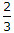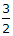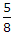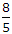Explanation:
No answer description is available. Let's discuss.

28.
The maximum deflection of a simply supported beam of span L, carrying an isolated load at the centre of the span ; flexural rigidity being EI, is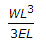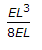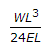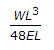Explanation:
No answer description is available. Let's discuss.

29.
The strain energy due to volumetric strain
is directly proportional to the volume
is directly proportional to the square of exerted pressure
is inversely proportional to Bulk modulus
all the above.
If the strain energy stored per unit volume in a hollow shaft subjected to a pure torque when t attains maximum shear stress fs the ratio of inner diameter to outer diameter, is